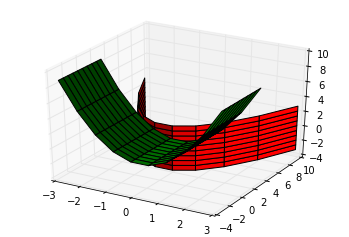In :
%matplotlib qt

In :
import numpy as np
import matplotlib.pyplot as pt


Consider the three equations:

$$y=x^2+\delta$$ $$z=x^2-\delta$$ $$y=z^2+\delta$$

In :
delta = 0.5

In :
from mpl_toolkits.mplot3d import Axes3D
fig = pt.figure()

res = 10j

x, z = np.mgrid[-3:3:res,-3:3:res]
y = x**2 + delta

ax.plot_surface(x, y, z, color="red", cstride=1, rstride=1)

if 1:
y, x = np.mgrid[-3:3:res,-3:3:res]
z = x**2 - delta

ax.plot_surface(x, y, z, color="green", cstride=1, rstride=1)

if 0:
x, z = np.mgrid[-3:3:res,-3:3:res]
y = z**2 + delta

ax.plot_surface(x, y, z, color="blue", cstride=1, rstride=1)Need better plotting tool. See corresponding three-quadratics.py.